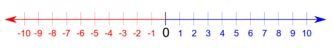## LetsPlayMaths.Com

WELCOME TO THE WORLD OF MATHEMATICS

# Class 5 Integer

Integers

Absolute value of an Integer

Comparison of integers

Addition of Positive and Negative Integers

Subtraction of Integers

Integer Test

Integer Worksheet

## Integers

When positive numbers, negative numbers and zero taken together are known as integers.

-4, -3, -2, -1, 0, +1, +2, +3, +4, ... are all integers.

+1, +2, +3, +4, ... known as positive integers and written as 1, 2, 3, 4, ...

-4, -3, -2, -1, ... known as negative integers.

0 is an integer but neither positive nor negative.

## Absolute value of an Integer

As we know 5 and -5 are same distance from 0. Both the integers are 5 units away from 0. The unit irrespective of the sign of the numbers is called absolute value.

Thus, the absolute value of 5 and -5 is 5. Two bars II stand for absolute value of the number written between them.

It is denoted as I 5 I = 5 and I -5 I = 5

Example 1. Find the absolute value of -8.

Solution. l -8 l = 8

Example 2. Find the absolute value of +15.

Solution. l +15 l = 15

## Comparison of integersNumber on the right is greater than the number on the left.

7 is on the right of 3, hence 7 > 3.
-2 is on the right of -9, hence -2 > -9.

When two positive integers are added, first add both the numbers and positive sigh is given for the sum. Let’s have a look at some example.

Example 1. Add +3 and +5.

Solution. (+3) + (+5) = +8.

Example 2. Add +9 and +11.

Solution. (+9) + (+11) = +20.

When two negative integers are added, first add both the numbers and negative sign is given for the sum. Let’s have a look at some example.

Example 1. Add -5 and -7.

Solution. (-5) + (-7) = -12.

Example 2. Add -25 and -15.

Solution. (-25) + (-15) = -40.

## Addition of Positive and Negative Integers

One positive and one negative number are added by subtracting them and giving the sign of the greater number to the result. Let’s have a look at some example.

Example 1. Add + 15 and -3.

Solution. (+15) + (-3) = +12.

Example 2. Add -16 and +8.

Solution. (-16) + (+8) = -8.

## Subtraction of Integers

Subtraction of integer is nothing but adding the minuend and subtrahend by changing the sign of the subtrahend. Let’s have a look at some examples.

Example 1. Subtract +12 from +18.

Solution. (+18) - (+12)
Keep minuend same, change the sign of subtrahend and then add.
(+18) + (-12)
Now, we have one positive and one negative number. We have to subtract and then give bigger number sign.
So, the result will be +6.

Integer Test- 1

Integer Test- 2

## Integer Worksheet

Integer Worksheet- 1

Integer Worksheet- 2

Integer Worksheet- 3

Integer Worksheet- 4